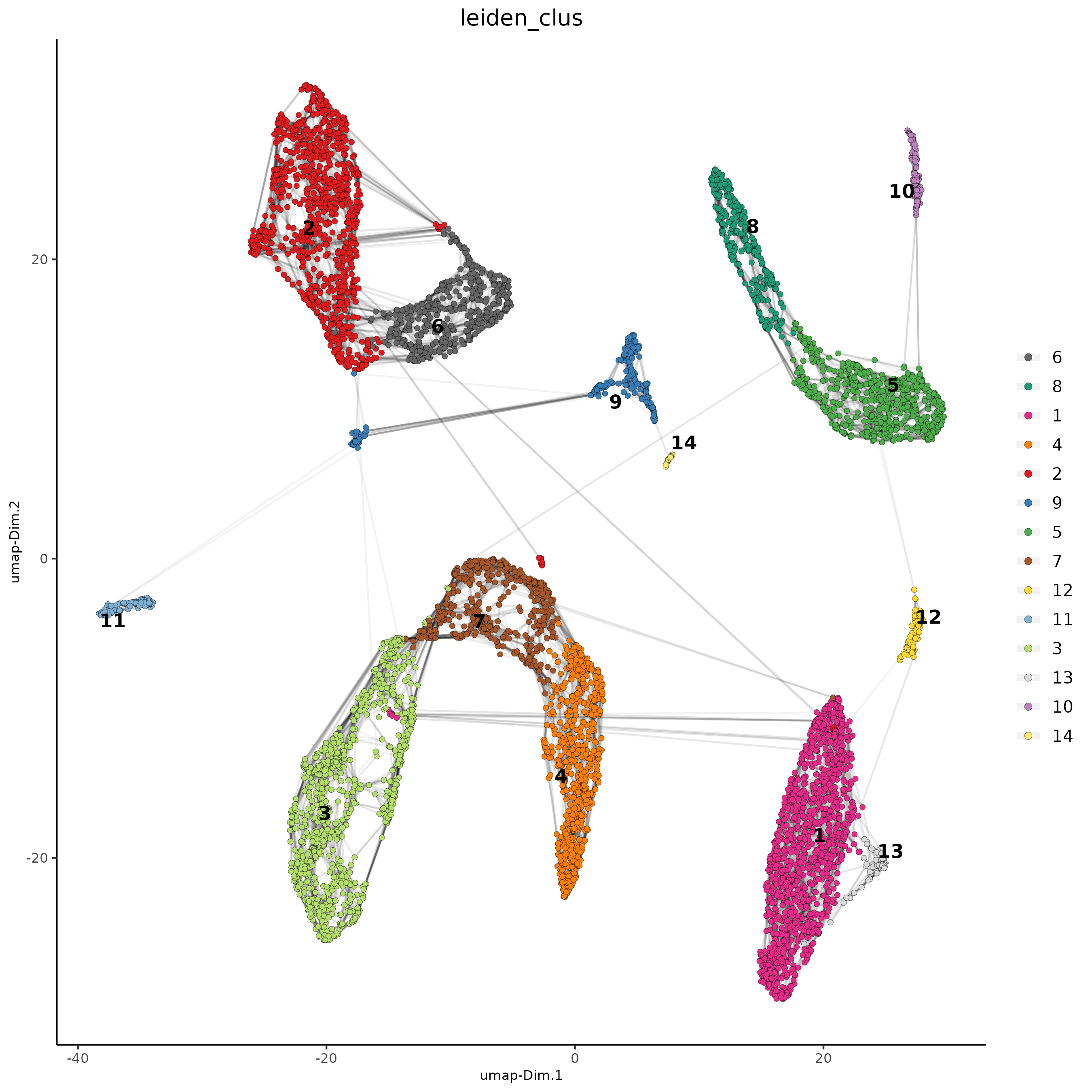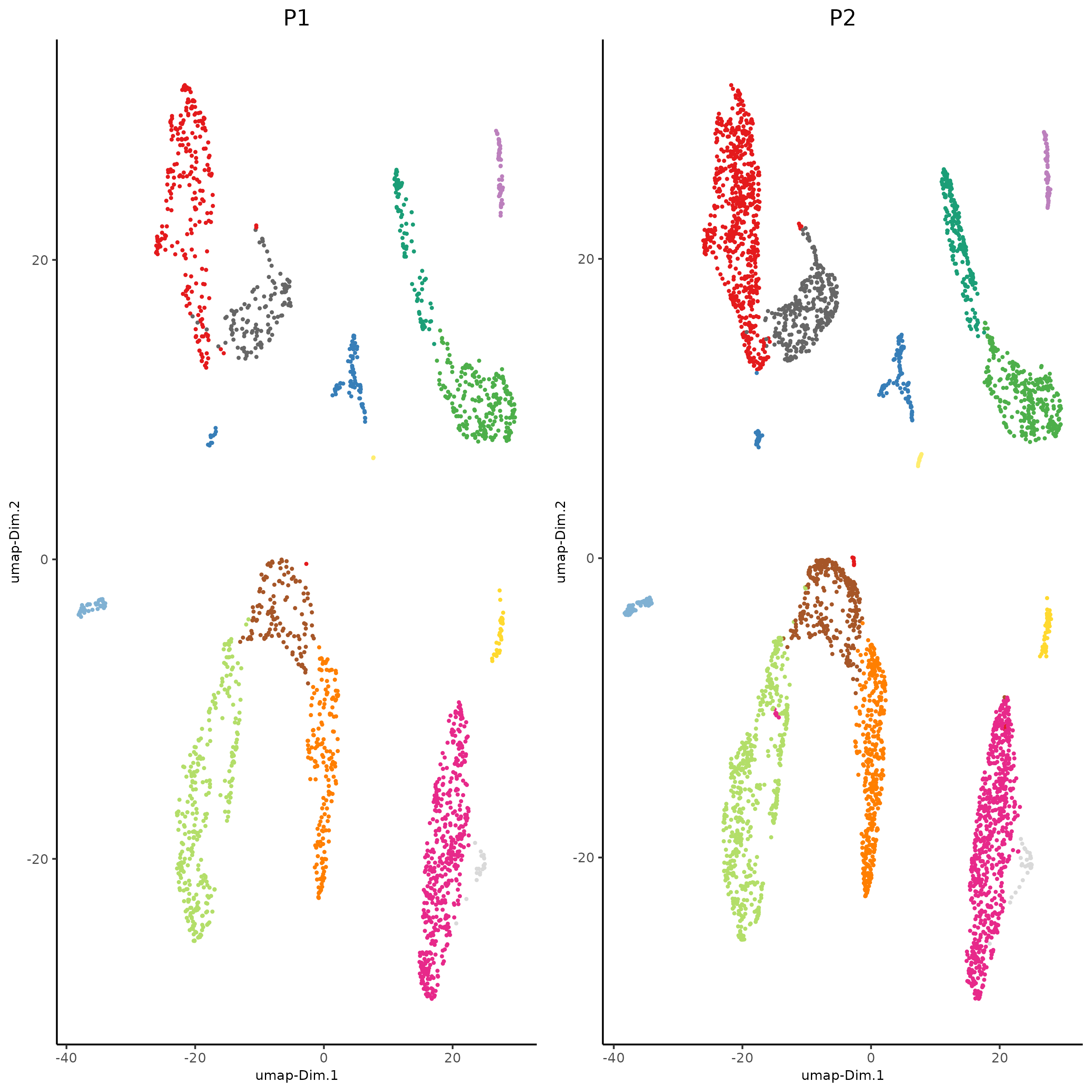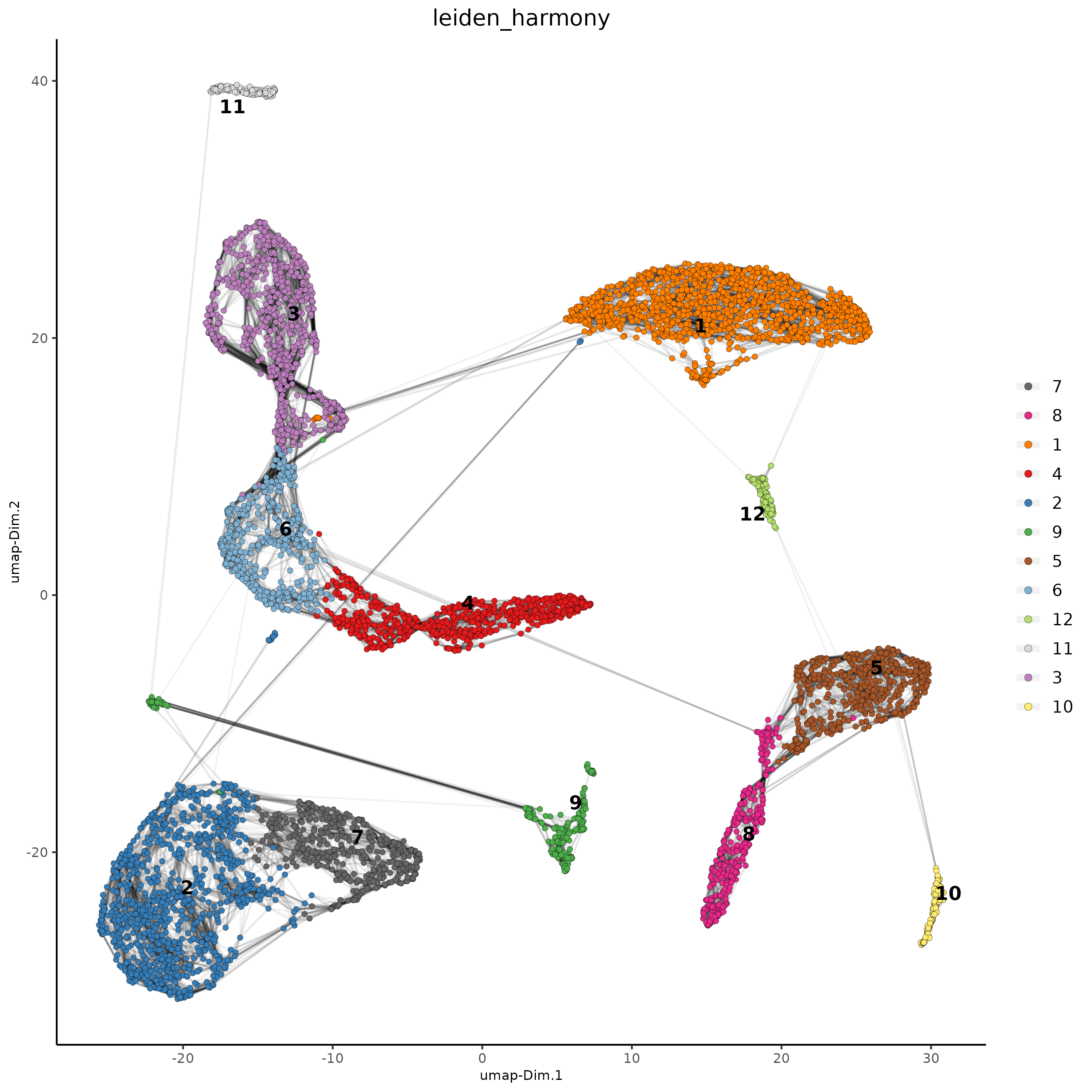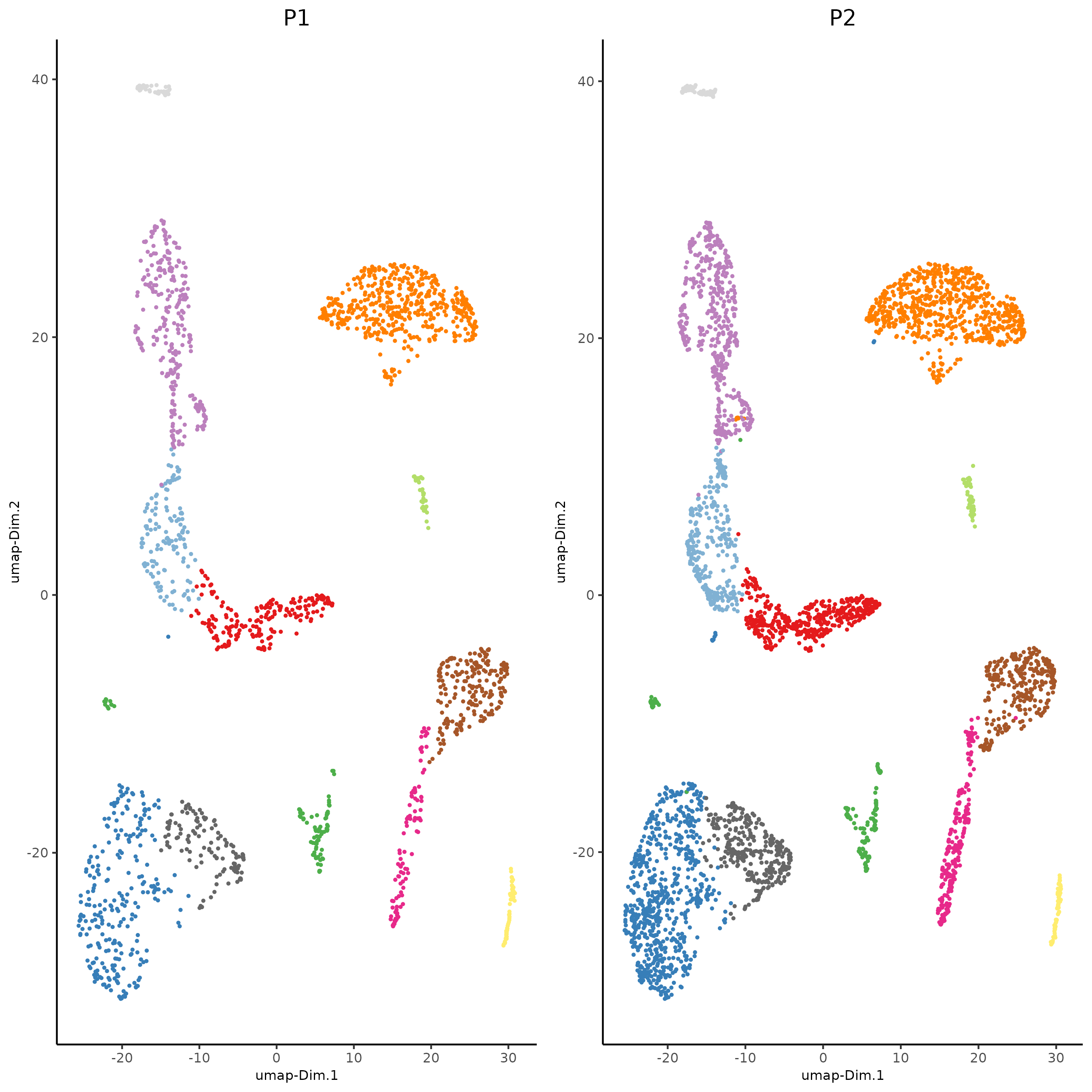# Integration of single cell datasets#

Date:

2022-09-16

```# Ensure Giotto Suite is installed.
if(!"Giotto" %in% installed.packages()) {
devtools::install_github("drieslab/Giotto@suite")
}

# Ensure GiottoData, a small, helper module for tutorials, is installed.
if(!"GiottoData" %in% installed.packages()) {
devtools::install_github("drieslab/GiottoData")
}
library(Giotto)
# Ensure the Python environment for Giotto has been installed.
genv_exists = checkGiottoEnvironment()
if(!genv_exists){
# The following command need only be run once to install the Giotto environment.
installGiottoEnvironment()
}
```

## Set up Giotto Environment#

```library(Giotto)
library(GiottoData)

# 1. set working directory
results_folder = 'path/to/result'

# Optional: Specify a path to a Python executable within a conda or miniconda
# environment. If set to NULL (default), the Python executable within the previously
# installed Giotto environment will be used.
my_python_path = NULL # alternatively, "/local/python/path/python" if desired.

# 2. create giotto instructions
instrs = createGiottoInstructions(save_dir = results_folder,
save_plot = TRUE,
show_plot = FALSE,
python_path = my_python_path)
```

## Dataset explanation#

This is a tutorial for Harmony integration of different single cell RNAseq datasets using two prostate cancer patient datasets. Ma et al. Processed 10X Single Cell RNAseq from two prostate cancer patients. The raw dataset can be found here

## Part 1: Create Giotto object from 10X dataset and join#

```giotto_P1<-createGiottoObject(expression = get10Xmatrix("path/to/P1_result/outs/filtered_feature_bc_matrix",
gene_column_index = 2,
remove_zero_rows = TRUE),
instructions = instrs)

giotto_P2<-createGiottoObject(expression = get10Xmatrix("path/to/P2_result/outs/filtered_feature_bc_matrix",
gene_column_index = 2,
remove_zero_rows = TRUE),
instructions = instrs)

giotto_SC_join = joinGiottoObjects(gobject_list = list(giotto_P1, giotto_P2),
gobject_names = c('P1', 'P2'),
join_method = "z_stack")
```

## Part 2: Process Joined object#

```giotto_SC_join <- filterGiotto(gobject = giotto_SC_join,
expression_threshold = 1,
feat_det_in_min_cells = 50,
min_det_feats_per_cell = 500,
expression_values = c('raw'),
verbose = T)

## normalize
giotto_SC_join <- normalizeGiotto(gobject = giotto_SC_join,
scalefactor = 6000)

## add gene & cell statistics
expression_values = 'raw')
```

## Part 3: Dimension reduction and clustering#

```## PCA ##
giotto_SC_join <- calculateHVF(gobject = giotto_SC_join)
giotto_SC_join <- runPCA(gobject = giotto_SC_join,
center = TRUE,
scale_unit = TRUE)
# Check screeplot to select number of PCs for clustering
# screePlot(giotto_SC_join, ncp = 30, save_param = list(save_name = '3_scree_plot'))

## WITHOUT INTEGRATION ##
# --------------------- #

## cluster and run UMAP ##
# sNN network (default)
showGiottoDimRed(giotto_SC_join)
giotto_SC_join <- createNearestNetwork(gobject = giotto_SC_join,
dim_reduction_to_use = 'pca',
dim_reduction_name = 'pca',
dimensions_to_use = 1:10,
k = 15)

# Leiden clustering
giotto_SC_join <- doLeidenCluster(gobject = giotto_SC_join,
resolution = 0.2,
n_iterations = 1000)

# UMAP
giotto_SC_join = runUMAP(giotto_SC_join)

plotUMAP(gobject = giotto_SC_join,
cell_color = 'leiden_clus',
show_NN_network = T,
point_size = 1.5,
save_param = list(save_name = "4_cluster_without_integration"))
``````dimPlot2D(gobject = giotto_SC_join,
dim_reduction_name = 'umap',
point_shape = 'no_border',
cell_color = "leiden_clus",
group_by = "list_ID",
show_NN_network = F,
point_size = 0.5,
show_center_label = F,
show_legend =F,
save_param = list(save_name = "4_list_without_integration"))
```Harmony is a integration algorithm developed by Korsunsky, I. et al.. It was designed for integration of single cell data but also work well on spatial datasets.

```## WITH INTEGRATION ##
# --------------------- #

## data integration, cluster and run UMAP ##

# harmony
#library(devtools)
#install_github("immunogenomics/harmony")
library(harmony)

giotto_SC_join = runGiottoHarmony(giotto_SC_join,
vars_use = 'list_ID',
do_pca = F)

## sNN network (default)
#showGiottoDimRed(giotto_SC_join)
giotto_SC_join <- createNearestNetwork(gobject = giotto_SC_join,
dim_reduction_to_use = 'harmony',
dim_reduction_name = 'harmony',
name = 'NN.harmony',
dimensions_to_use = 1:10,
k = 15)

## Leiden clustering
giotto_SC_join <- doLeidenCluster(gobject = giotto_SC_join,
network_name = 'NN.harmony',
resolution = 0.2,
n_iterations = 1000,
name = 'leiden_harmony')

# UMAP dimension reduction
#showGiottoDimRed(giotto_SC_join)
giotto_SC_join = runUMAP(giotto_SC_join,
dim_reduction_name = 'harmony',
dim_reduction_to_use = 'harmony',
name = 'umap_harmony')

plotUMAP(gobject = giotto_SC_join,
dim_reduction_name = 'umap_harmony',
cell_color = 'leiden_harmony',
show_NN_network = T,
point_size = 1.5,
save_param = list(save_name = "4_cluster_with_integration"))
``````dimPlot2D(gobject = giotto_SC_join,
dim_reduction_name = 'umap_harmony',
point_shape = 'no_border',
cell_color = "leiden_harmony",
group_by = "list_ID",
show_NN_network = F,
point_size = 0.5,
show_center_label = F,
show_legend =F ,
save_param = list(save_name = "4_list_with_integration"))
```